## Material Science/States of Matter

The study of material science involves investigating the relationships that exist between the structures and properties of matter. Several specific areas, that are related to traditional physics courses, will be isolated and studied from the material science and engineering discipline. Further investigation of this extensive subject area is encouraged. This topic is associated with chapter 9 of College Physics by Serway and Faughn.

 Definitions matter moduli material terms #1 stress strain Experiments rubber band aluminum concrete terms #2 problems competencies

Core Component/Definitions
MATTER is divided into four categories: solids (crystalline and amorphous), liquids, gases, plasma, then fluids

MATERIALS can be conveniently grouped into three basic classifications, primarily based on chemical makeup and atomic structure.

METALS - metallic materials with a large number of non localized electrons (not bound to particular atoms) which contribute to their properties of conductivity, heat exchange, and strength. (example - Iron)

CERAMICS - compounds between metallic and nonmetallic elements. most frequently oxides, nitrides, and carbides. Characteristics include : generally hard but very brittle and insulative to the passage of heat and electricity. ( example - glass)

POLYMERS - include the plastic and rubber materials. Many are organic and typically have very large molecular structures. (example - polyethylene toys)

Two additional groups of engineering materials are:

COMPOSITES - materials consisting of more than one material type. (example - fiberglass ; glass fibers embedded within a polymeric material)

SEMICONDUCTORS - have electrical properties intermediate between conductors and insulators. (example - computer chip made of silicon)

STRESS
Stress
occurs in a material when there are external and internal forces in equilibrium. The quantification of stress can be called TENSILE STRESS, COMPRESSIVE STRESS, AND SHEAR STRESS. All are expressed as force per unit area. Where σ is stress (in Pascals - Pa), F is force (in Newtons), and A is cross-sectional area (in m2).
σ= F / A

THREE TYPES OF STRESS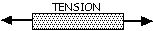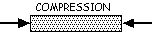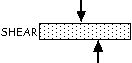STRAIN
Strain (ε) is the measure of deformation in a given material. The quantification of strain is referred to as TENSILE STRAIN which is the ratio of a change in length (ΔL) to the original length ( LO ).
ε = Δ L / LO
Shear strain is the ratio of the change in horizontal distance / height. (ε = Δx/h) , as diagramed below.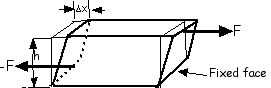MODULI
The ratio of stress to strain creates a modulus. For example, tensile stress to tensile strain is termed YOUNG'S MODULUS (Y) or ELASTIC MODULUS. This quantity is used to characterize a material under either tension or compression and only depends on the material itself.
In equation form: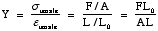Y is measured in PASCALS (Pa) and is a constant for a given material.
example values: Iron = 100 x 109 N/m2 Nylon = 5 x 109 N/m2
An additional deformation may occur when equal and opposite forces are applied across an objects opposite faces. This shear stress produces it own proportionality constant referred to as SHEAR MODULUS.
We define the Shear Modulus as the shear stress divided by the shear strain.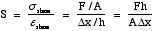Shear Modulus (S) is measured in Pascals (Pa). example : Iron = 40 x 109 N/m2
One final deformation involves the compression of an object by applying force at right angles to all of its faces. These forces now generate VOLUME STRESS and VOLUME STRAIN, the ratio of change in volume to the original volume. This new ratio is defined as BULK MODULUS (B) . Where: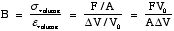Bulk Modulus (B) is also measured in Pascals (Pa). example: Iron = 90 x 109 N/m2

Several additional concepts that support the study of stress and strain are listed below.
FRACTURE - the amount of stress (tensional, compressional, or shear) on a solid that will produce breakage of that object.
ELASTIC LIMIT - the point where a solid will return to its original shape under a given applied force.
HOOKE’S LAW - when strain is proportional to stress a material will obey this law.
PLASTIC REGION - an object enters this region when the material does not return to its original shape when an applied force is removed.

DUCTILITY - when a material is drawn out in tension without fracturing. Ductile materials are strong and plastic.
 MALLEABILITY - permanently extending an object in all directions without fracture by hammering or pressing. Materials must be plastic, but not so dependent on strength. TOUGHNESS - the amount of energy that a material can absorb before it fractures. BRITTLENESS - a material that breaks with little permanent distortion. It lacks toughness and ductility. HARDNESS - the resistance to wear or indentation of a material.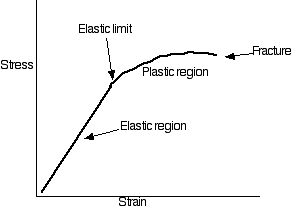II. Experimental Component
EXPERIMENT #1 Tensile Strength of rubber band
Record the thickness ______ (mm) and length _______ (mm) of the specimen.
Attach a mass to the rubber band to stretch it. Measure the length. Calculate the weight of the mass hanging on the rubber band.
Add mass in small steps repeating the previous step each time.
Continue this until the rubber band breaks.
Graph stress vs strain
EXPERIMENT #2 Tensile Test of an Aluminum Cylinder.
• Make measurements of the aluminum sample recording each of the characteristics.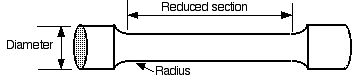• Mount the sample in the tensile testing machine. Insert one end into the load cell and the other end in the moving crosshead. (fig. 1)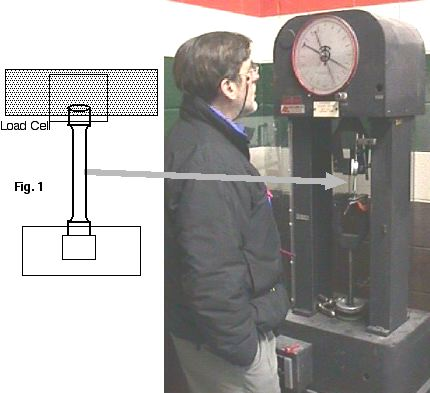• Setup the extensometer to measure the sample elongation.
• CAUTION !! Close the protective shield door before applying any force to the sample.
• Apply force slowly and record the values from the force gauge and the extensometer until fracture of the sample occurs.
• Calculate the tensile stress and tensile strain. Calculate Young’s modulus.
• If sufficient readings were obtained, graph stress versus strain and identify the salient features of this characteristic curve.
EXPERIMENT #3 Compression Test of a Concrete sample.
• Using the pre-made concrete sample provided determine its outer dimensions and mass. Also calculate the volume and density of the sample.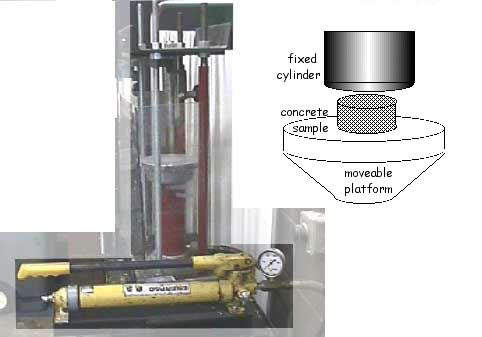• Place the sample in the compression tester. WEAR PROTECTIVE EYEGLASSES!
• Close the bleeder valve on the hydraulic pump.
• Lower the hydraulic cylinder, using the pump handle, until contact with the sample is made. (ensure that contact areas above and below the sample completely cover the samples surface)
• Continue to apply pressure to the sample until fracture occurs. At the point of fracture make note of the pressure reading on the pump gauge. (requires two people)
• Compute the compressive stress on the sample at maximum pressure. (hint - remember you are reading pressure in pounds per square inch (psi) and you must convert to metric force per unit area)
• Computing the compressive strain for a concrete sample is difficult to do accurately. State why this is so.
• Compute the force acting on the concrete sample.

III. Exercises

1) Determine the pressure of:

• a) a bicycle (10.0 kg) with a 62.0-kg rider. Each tire is in contact with the ground in a shape that is 1.0 cm by 4.0 cm.
• b) a 42-kg girl has a pair shoes that spreads her weight over 15 cm2 for each shoe.
• c) The same girl when wearing a pair of stiletto heels that allows her to put all of her weight onto 1 cm2 on each shoe.
If the girl in part c is standing on a concrete floor, what kind of stress, and how much, is created?

2) Complete the following problems from chapter 9, College Physics (Serway, Faughn)
Problems (beginning on page 295) # 1, 2, 3, 5, 6, 8 and 14.

 problems 3-5 are taken from Materials Science and Engineering, third edition, by William Callister 3) From the tensile stress-strain behavior in the graph for a brass specimen calculate: (a) Young's Modulus (b) The maximum load (in newtons) that can be sustained by a cylindrical specimen having an original diameter of 12.8 mm. (c) The change in length of a specimen originally 254 mm long which is subjected to a tensile stress of 50,000 psi (345 MPa). click on the graph to see the smaller section in more detail (which is a magnified version of the left-most section of the graph)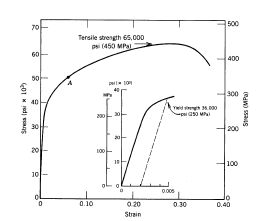4) The pressure at a depth of 300m in the ocean exceeds sea-level atmospheric pressure by 2.94 x 106 Pa. By how much does the volume of a 1000cm3 aluminum object contract when it is lowered to this depth?

5) The reciprocal of the bulk modulus B of a liquid is called its compressibility k, so that k=1/B. The bulk modulus of water is 2.3 x 109 Pa. (a) Find its compressibility per atmosphere of pressure, where 1 atm = 1.013 X 105 Pa is the pressure exerted by the earth's atmosphere at sea level. (b) How much pressure in atm is needed to compress a sample of water by 0.1% ?

 COMPETENCIES for Governor's School Physics 2004-5 using College Physics by Serway and Faughn, sixth edition 2003 and Material Science and Engineering by Callister, third edition 1994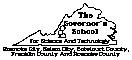COMPETENCY 25
Develop a beginning model for material science that includes the relationships that exist between the structures and properties of matter by being able to:
ENABLING OBJECTIVES
25.1 Distinguish between the three basic groupings of materials: metals, ceramics, and polymers.
25.2 State the relationship of forces for the stresses of tension, compression, and shear.
25.3 Explain the difference between stress and strain.
25.4 Calculate tensile stress and strain.
25.5 Compute Young’s modulus and explain when this is an appropriate measurement of a solid.
25.6 Calculate Bulk modulus and explain under what physical conditions you may study this factor.
25.7 Diagram and interpret a stress versus strain graph for a solid. Include elastic region, elastic limit, fracture, and plasticity zone.
25.8 Calculate Shear modulus and diagram the forces and displacements utilized in its measurement.
25.9 Describe the terms ductility, malleability, toughness, brittleness and hardness in terms of the three general groupings of materials.